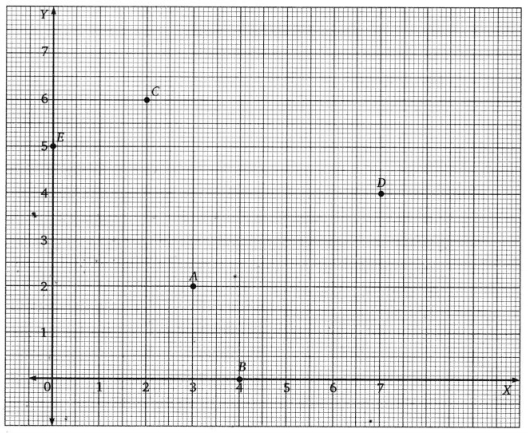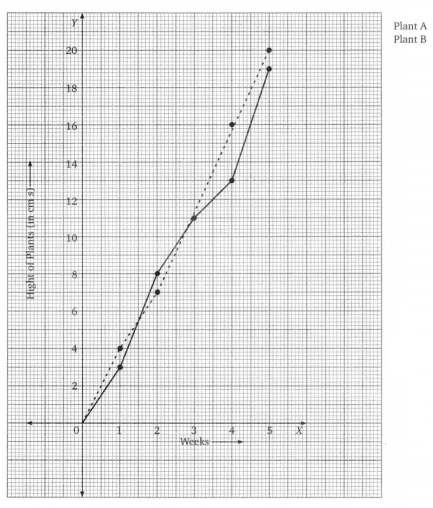# Grade 8 Introduction to Graphs Worksheets

## Grade 8 Maths Introduction to Graphs Multiple Choice Questions (MCQs)

1. The coordinate of A in the adjacent graph is:
(a) (-7, 3)
(b) (7, -7)
(c) (-6, -1)
(d) (2, -3)

2. The coordinate of B in the adjacent graph is:
(a) (-7, 3)
(b) (7, -7)
(c) (-6, -1)
(d) (2, -3)

3. The coordinate of C in the adjacent graph is:
(a) (-7, 3)
(b) (7, -7)
(c) (-6, -1)
(d) (2, -3)

4. The coordinate of D in the adjacent graph is:
(a) (-7, 3)
(b) (7, -7)
(c) (-6, -1)
(d) (2, -3)5. The coordinate of E in the above graph is:
(a) (9, -3)
(b) (-4, 2)
(c) (-3, -4)
(d) (-7, 9)

6. If y – coordinate of a point is zero, then this point always lies:
(c) x-axis
(d) y-axis

7. If x – coordinate of a point is zero, then this point always lies:
(c) x-axis
(d) y-axis

8. The point (-4, -3) means:
(a) x = -4, y = -3
(b) x = -3, y = -4
(c) x = 4, y = 3
(d) None of these

9. On joining points (0, 0), (0, 2), (2, 2) and (2, 0) we obtain a:
(a) Square
(b) Rectangle
(c) Rhombus
(d) Parallelogram

10. Point (-2, 3) lies in the:

11. Point (0, -2) lies:
(a) on the x-axis
(c) on the y-axis

12. Abscissa of the all the points on x-axis is:
(a) 0
(b) 1
(c) -1
(d) any number

13. Ordinate of the all the points on x-axis is
(a) 0
(b) 1
(c) -1
(d) any number

14. Ordinate of the all the points on y-axis is
(a) 0
(b) 1
(c) -1
(d) any number.

15. The point whose ordinate is 4 and which lies on y-axis is:
(a) (4, 0)
(b) (0, 4)
(c) (1, 4)
(d) (4, 2)

16. The perpendicular distance of the point P(3, 4) from the y-axis is:
(a) 3
(b) 4
(c) 5
(d) 7

17. The number of coordinate axis is:
(a) 1
(b) 2
(c) 4
(d) 3

18. The y-axis is a:
(a) horizontal line
(b) vertical line
(c) oblique line
(d) vertical seqment

19. The abscissa of the point (-3, -2) is
(a) -3
(b) -2
(c) -5
(d) -1

20. Y-coordinate is also called:
(a) Abscissa
(b) Ordinate
(c) y-axis
(d) None of these

### Class 8 Maths Introduction to Graphs Fill In The Blanks

1. The point where X-axis and Y-axis meet is called ……………….. .
2. Who is considered to be father of cartesian system …………….. .
3. The X-coordinate of the point A (3, 7) is ……………… .
4. Perpendicular distance of the point (2, 3) from X-axis is …………….. .
5. The Y-coordinate of the point B (4, 1) is ………………… .
6. Perpendicular distance of the point (5, 2) from Y-axis is ……………… .
7. Point (-6, 4) lies in the …………… quadrant.
8. Point (0,4) lies in the ……………… quadrant.
9. Point (5,0) lies in the ………………. quadrant.
10. Abscissa of all the points on y-axis is ………………. .

From the given graph, locate the position of points A, B, C, D and E.For an experiment in science, Vikram and Rohit grew one plant each under similar lab conditions. Their heights were measured at the end of each week for 5 weeks, the result is shown by the follow:

1. Write height of plant A and plant B after
(a) 2 weeks
(b) 5 weeks.
2. Write difference of height of the two plants after 5 weeks.
3. During which week plant A grew most?
4. Druing which week plant B grew least?The quantity of petrol filled in a car and the cost of petrol are given in the following table:1. Draw the graph of above data find the cost 12 l of petrol using graph. How much petrol can be purchased for ₹ 800?